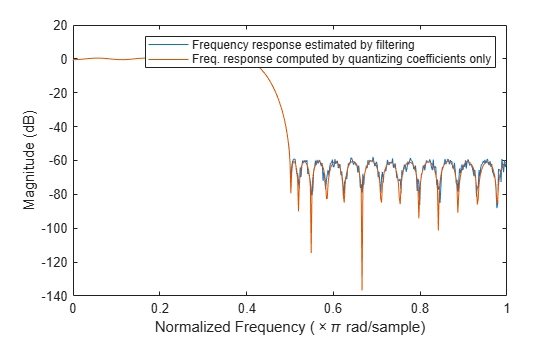Documentation

# freqrespest

Frequency response estimate via filtering

## Syntax

[h,w] = freqrespest(sysobj,L) [h,w] = freqrespest(sysobj,L,param1,value1,param2,value2,...) [h,w] = freqrespest(sysobj,L,opts) freqrespest(sysobj,...) 

## Description

[h,w] = freqrespest(sysobj,L) estimates the frequency response of a filter System object™, sysobj. A set of input data is filtered and then forming the ratio between output data and input data. The test input data comprises sinusoids with uniformly distributed random frequencies.

Use this technique for comparing the performance of fixed-point filters to that of another filter type. You can, for example, compare fixed—point frequency response estimate to that of a similar filter that uses quantized coefficients, but applies floating-point arithmetic internally. Such comparison determines whether the fixed-point filter performance closely matches the floating-point, quantized coefficients version of the filter.

L is the number of trials to use to compute the estimate. If you do not specify this value, L defaults to 10. More trials generates a more accurate estimate of the response, but require more time to compute the estimate.

h is the estimate of the complex frequency response. w contains the vector of frequencies at which h is estimated.

[h,w] = freqrespest(sysobj,L,param1,value1,param2,value2,...) uses parameter value (PV) pairs as input arguments to specify optional parameters for the test. These parameters are the valid PV pairs. Enter the parameter names as input arguments in single quotation marks. The following table provides valid parameters for [h, w].

Parameter Name

Default Value

Description

NFFT

512

Number of FFT points to use.

NormalizedFrequency

true

Indicate whether to use normalized frequency or linear frequency. Values are true (use normalized frequency), or false (use linear frequency). When you specify false, you must supply the sampling frequency Fs.

Fs

normalized

Specify the sampling frequency when NormalizedFrequency is false. No default value. You must set NormalizedFrequency to false before setting a value for Fs.

SpectrumRange

half

Specify the spectrum range to be used as whole or half (default).

CenterDC

false

Specify whether to set the center of the spectrum to the DC value in the output plot. If you select true, both the negative and positive values appear in the plot. If you select false, DC appears at the origin of the axes.

ArithmeticARITHAnalyze the filter System object, based on the arithmetic specified in the ARITH input. ARITH can be set to double, single, or fixed. The analysis tool assumes a double-precision filter when the arithmetic input is not specified and the filter System object is in an unlocked state.

freqrespest requires knowledge of the input data type. Analysis cannot be performed if the input data type is not available. If you do not specify the Arithmetic parameter, i.e., use the syntax [h,w] = freqrespest(sysobj) , then the following rules apply for this method:

• The System object state is Unlockedfreqrespest performs double precision analysis.

• The System object state is Lockedfreqrespest performs analysis based on the locked input data type.

When you do specify the Arithmetic parameter, i.e., use the syntax [h,w] = freqrespest(sysobj,'Arithmetic', ARITH), review the following rules for this method. Which rule applies depends on the value you set for the Arithmetic parameter.

ValueSystem Object StateRule
ARITH = 'double'Unlockedfreqrespest performs double-precision analysis.
Lockedfreqrespest performs double-precision analysis.
ARITH = 'single'Unlockedfreqrespest performs single-precision analysis.
Lockedfreqrespest performs single-precision analysis.
ARITH = 'fixed'Unlockedfreqrespest produces an error because the fixed-point input data type is unknown.
LockedIf the input data type is double or single, then freqrespest produces an error because the fixed-point input data type is unknown.
When the input data is of fixed-point type, freqrespest performs analysis based on the locked input data type.

The following Filter System objects are supported by this analysis function:

[h,w] = freqrespest(sysobj,L,opts) uses an object, opts, to specify the optional input parameters. This specification is not done directly by specifying PV pairs as input arguments. Create opts with

opts = freqrespopts(sysobj);

Because opts is an object, you use set to change the parameter values in opts before you use it with freqrespest. For example, you could specify a new sample rate with

set(opts,'fs',48e3); % Same as opts.fs=48e3

freqrespest(sysobj,...) with no output argument launches FVTool.

freqrespest can also compute the frequency response of double-precision floating filters. Such filters cannot be converted to transfer-function form without introducing significant round off errors which affect the freqz frequency response computation. Examples of these kinds of filters include state-space or lattice filters, especially if they are high-order filters.

## Examples

collapse all

This example shows to how to estimate the frequency response of a fixed-point FIR filter.

firFilt = design(fdesign.lowpass(.4,.5,1,60),'equiripple','Systemobject',true); dataIn = fi(randn(16,15),1,16,15); dataOut = firFilt(dataIn); %#ok [h,w] = freqrespest(firFilt); %#ok % This should be about the same as freqz. release(firFilt);

Continuing with filter firFilt, change FullPrecisionOverride to false and then specify the word lengths and precision (the fraction lengths) applied to the output from internal addition and multiplication operations. After you set the word and fraction lengths, use freqrespest to compute the frequency response estimate for the fixed-point filter.

firFilt.FullPrecisionOverride = false; firFilt.ProductDataType = 'Custom'; firFilt.CustomProductDataType = numerictype(1,16,15); firFilt.AccumulatorDataType = 'Custom'; firFilt.CustomAccumulatorDataType = numerictype(1,16,15); firFilt.OutputDataType = 'Same as accumulator'; dataOut = firFilt(dataIn); [h,w] = freqrespest(firFilt,2); [h2,w2] = freqz(firFilt,512); plot(w/pi,20*log10(abs([h,h2]))) legend('Frequency response estimated by filtering',... 'Freq. response computed by quantizing coefficients only'); xlabel('Normalized Frequency (\times\pi rad/sample)') ylabel('Magnitude (dB)')